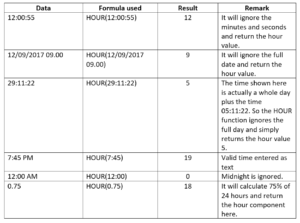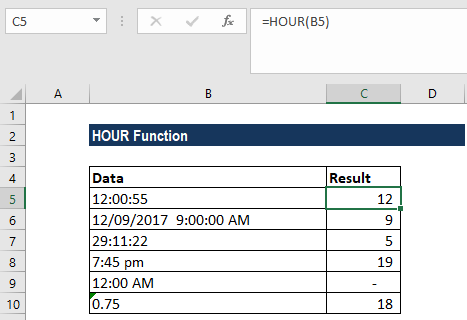# HOUR Function

Returns the hour component of a time

## What is the HOUR Function?

The HOUR function is categorized under Excel DATE/TIME functions. The function returns the hour component of a time as a number between 0-23. For example, it will return 13 for 1:49 PM.

### Formula

=HOUR(serial_number)

This function uses only one argument:

Serial_number (required argument) – The time that contains the hour we want to find. It will return the hour of the time value. The hour given will be an integer between 0 to 23 where 0 represents 12:00 AM and 23 represents 11:00 PM.

The argument can be entered as:

1. A decimal (serial number) representation of an Excel time
2. A text representation of a time
3. A reference to a cell containing a time
4. A time value returned from another Excel function or formula

### How to use the HOUR Function in Excel?

As a worksheet function, the HOUR function can be entered as part of a formula in a cell of a worksheet. To understand the uses of the function, let’s consider an example:

#### Example 1

Let’s see how this function work when we have provided the following inputs:The results in Excel are shown in the screenshot below:In case we get an error, we need to remember that Excel stores dates and times as serial numbers. For example, the date January 1, 2000, 12:00 PM is equal to the serial number 32526.5 in Excel. To check that Excel correctly recognizes a date or time, we can temporarily format the date as a number.

### A few things to remember about the HOUR Function

1. Time values are a portion of a date value and represented by a decimal number (for example, 12:00 PM is represented as 0.5 because it is half of a day).
2. #VALUE! error – Occurs when the given serial_number is not a valid Excel time.

Thanks for reading CFI’s guide to important Excel functions! By taking the time to learn and master these functions, you’ll significantly speed up your financial analysis. To learn more, check out these additional CFI resources:

• Excel Functions for Finance